# MathAddons+ || Useful functions all in one place!Model || V1

MathAddons+ is a simple yet large module for all your mathematical needs
With over 70 functions, such as calculating the mean, scientific notation, fraction conversion, and more!

Setting the Module Up

Using any of the functions is simple:

``````local Math = require(game.ReplicatedStorage.MathAddons)
local numbers = {1,2,3,4,5,6}
local average = Math.avg(numbers)
print(average) -- 3.5
``````

Keep in mind that some functions aren’t as beginner-friendly as coin flip and average, functions such as integration and base conversion require reading the documentation below to understand what each parameter represents.

Most Recent Update

FAQ

? What does MathAddons bring that regular math functions don’t?

• ! This module has 50 math functions that aren’t seen in the regular math library.

? Is there a noticeable performance difference between this module and the math library?

• ! I will say some functions such as integration do rely on loops, other than the functions rely on a great amount of looping, however, they won’t have a noticeable performance impact.

? Does this module get updated frequently?

• ! There is no specific schedule, it really depends on what I discover.

? Some of these functions are easy to make, why should I use them through the module?

• ! There isn’t any big motivation for this module to be used, working on this module is just a hobby to me

? I have a function suggestion! Where can I submit it?

• ! You can reach me by messaging me or replying to this post!

? Do I need credit to use this module in a public project?

• ! Credit is appreciated but absolutely not necessary!

Documentation

## Statistics and Probability

The functions that are usually used to calculate probabilities and make educated guesses.

Flip

Purpose: create a random chance
Parameters:

1. Number from 0-1, which represents a 0-100% chance

Output: Boolean
Explanation:

• If your chance is .5, you have the same chance of getting true as false. If your chance is .85, you are likely to return true, but uncertain. If your chance is .15, you are likely to return false.

Example:

``````local x = module.flip(.5) -- x is true or false due to a 50-50 chance
local y = module.flip(.98) -- y is likely going to be true due to a 98% chance
local z = module.flip(.01) -- z is most likely going to be false due to a 1% chance
``````
Standard Deviation

Purpose: Calculate the Standard Deviation of a Data Set
Parameters:

1. Table in the form of {a,b,c…} where all the values inside are numbers
2. Toggle the Population/Sample Deviation with a Boolean (OPTIONAL) || Defaulted to false

Output: Number
Explanation:

• This function returns a number representing a measure of how dispersed the data is about the mean. The larger it is, the less predictable the data set is.
I can leave some links below since I don’t fully understand this weird formula. The second parameter is optional and defaulted to false (Sample Deviation)

Standard deviation - Wikipedia
Example:

``````local x = module.sd({86,84,85,93,86}) -- x = 3.56370593624, this number is this high (meaning unpredictable) because of the outlier 93.
local y = module.sd({86,84,85,86}) -- y = 0.957427107756, much lower and predictable because there is no more outlier.
``````
Minimum

Purpose: return the smallest value in a table
Parameters:

1. Table in the form of {a,b,c…} where all the values inside are numbers

Output: Number
Explanation:

• Self-explanatory, this returns the smallest value from a table

Example:

``````local x = module.min({2,3,4,5,2,2,0,5}) -- x = 0 since its the smallest value.
local y = module.min({2,3,4,5,2,2,5}) -- y = 2 since 0 was removed and 2 is the next smallest number.
``````
Quartile 1

Purpose: returns the first quartile value of a data set
Parameters:

1. Table in the form of {a,b,c…} where all the values inside are numbers

Output: Number
Explanation:

• returns the number that’s 25% of the way in the dataset, a few more rules apply but this is a general idea

Example:

``````local x = module.q1({2,3,4,5,1,2,2,0,5}) -- x = 1.5
local y = module.q1({5,3,4,4,7,8,5,4,3,5,7,9}) -- y = 4
``````
Median

Purpose: returns the median of a data set
Parameters:

1. Table in the form of {a,b,c…} where all the values inside are numbers

Output: Number
Explanation:

• returns the middle number in a dataset (when sorted from least to greatest)

Example:

``````local x = module.median({2,3,4,5,1,2,2,0,5}) -- x = 2
local y = module.median({5,3,4,4,7,8,5,4,3,5,7,9}) -- y = 5
``````
Quartile 3

Purpose: returns the third quartile value of a data set
Parameters:

1. Table in the form of {a,b,c…} where all the values inside are numbers

Output: Number
Explanation:

• returns the number that’s 75% of the way in the dataset, a few more rules apply however, this is a general idea

Example:

``````local x = module.q3({2,3,4,5,1,2,2,0,5}) -- x = 4.5
local y = module.q3({5,3,4,4,7,8,5,4,3,5,7,9}) -- y = 7
``````
Maximum

Purpose: return the largest value in a table
Parameters:

1. Table in the form of {a,b,c…} where all the values inside are numbers

Output: Number
Explanation:

• Self-explanatory, this returns the largest value from a table

Example:

``````local x = module.max({2,3,4,5,2,2,0,5}) -- x = 5 since its the largest value.
local y = module.max({2,3,4,2,2,0}) -- y = 4 since 5 was removed and 4 is the next largest number.
``````
IQR

Purpose: returns the IQR of a data set
Parameters:

1. Table in the form of {a,b,c…} where all the values inside are numbers

Output: Number
Explanation:

• returns the difference of the third quartile to the first

Example:

``````local x = module.iqr({2,3,4,5,1,2,2,0,5}) -- x = 3
local y = module.iqr({5,3,4,4,7,8,5,4,3,5,7,9}) -- y = 3
``````
Range

Purpose: returns the range of a data set
Parameters:

1. Table in the form of {a,b,c…} where all the values inside are numbers

Output: Number
Explanation:

• returns the difference of the maximum to the minimum

Example:

``````local x = module.range({2,3,4,5,1,2,2,0,5}) -- x = 5
local y = module.range({5,3,4,4,7,8,5,4,3,5,7,9}) -- y = 6
``````
Mode

Purpose: returns the mode of a data set
Parameters:

1. Table in the form of {a,b,c…} where all the values inside are numbers

Output: Table of numbers
Explanation:

• returns which data value appears the most in the data set

Example:

``````local x = module.mode({2,3,4,5,1,2,2,0,5}) -- x = {2} (it appears 3 times)
local y = module.mode({5,3,4,4,7,8,5,4,3,5,7,9}) -- y = {5,4} (they appear the same amount of times)
``````

Purpose: returns the MAD of a data set
Parameters:

1. Table in the form of {a,b,c…} where all the values inside are numbers

Output: Number
Explanation:

• returns the average of the differences from each data value to the mean. the larger number shows that data values are far from the mean, meaning there is a large difference above and below the mean. A lower number means the values are closer together

Example:

``````local x = module.mad({2,3,4,5,1,2,2,0,5}) -- x = 1.4074074074074074
local y = module.mad({5,3,4,4,7,8,5,4,3,5,7,9}) -- y = 1.611111111111111
``````
Average

Purpose: returns the average of a data set
Parameters:

1. Table in the form of {a,b,c…} where all the values inside are numbers

Output: Number
Explanation:

• Adds up all the numbers in the data set and divides the sum by how many values there are

Example:

``````local x = module.avg({2,3,4,5,1,2,2,0,5}) -- x = 2.666666666666667
local y = module.avg({5,3,4,4,7,8,5,4,3,5,7,9}) -- y = 5.333333333333333
``````
Z Score

Purpose: returns the Z score of a dataset
Parameters:

1. Table in the form of {a,b,c…} where all the values inside are numbers

Output: Number
Explanation:

• The Z score is a value that represents how many standard deviations a data point is from the mean

Example:

``````local x = module.zscore({2,3,4,5,1,2,2,0,5}) -- x = {
["0"] = -1.539600717839002,
["1"] = -0.9622504486493764,
["2"] = -0.3849001794597506,
["3"] = 0.1924500897298751,
["4"] = 0.7698003589195008,
["5"] = 1.347150628109127}
local y = module.zscore({5,3,4,4,7,8,5,4,3,5,7,9},true) -- y = {
["3"] = -1.184755600676077,
["4"] = -0.6770032003863299,
["5"] = -0.1692508000965824,
["7"] = 0.8462540004829127,
["8"] = 1.35400640077266,
["9"] = 1.861758801062408}
``````
Linear Regression

Purpose: returns the function (or slope & y intercept) and correlation coefficient of a data set
Parameters:

1. As many data points as you please in the form of {x,y}
2. The last parameter would be a boolean to Toggle Function Format

Output: Function, Number (If Function Toggle is false: Number, Number, Number
Explanation:

• *Calculates the line of best fit, and the correlation coefficient (the number that represents how good the line matches the set.
Example:
``````local slope, yint, r = module.linreg({0,2},{1,2},{2,3},{5,9},{6,5},{4,7},{10,5})
-- slope = 0.4142857142857143
-- yint = 3.057142857142857
-- r = 0.5385164807134504
local func, r = module.linreg({1,2},{5,8},{4,5},true)
-- r = 0.9607689228305227
local predictedPoint = func(10) -- predictedPoint = 14.23076923076923
``````

## Miscellaneous

The math functions that don’t really fit a category

GCD

Purpose: returns the GCD of a data set
Parameters:

1. Number
2. Number

Output: Number
Explanation:

• Calculates the number which can divide evenly into both parameters

Example:

``````local x = module.gcd(45,10) -- x = 5
local y = module.gcd(63,14) -- y = 7
``````
LCM

Purpose: returns the LCM of a data set
Parameters:

1. Number
2. Number

Output: Number
Explanation:

• Calculates the smallest number which the two parameters can both divide into

Example:

``````local x = module.lcm(45,10) -- x = 90
local y = module.lcm(63,14) -- y = 126
``````
Floor

Purpose: rounds a number down to the nearest decimal (d)
Parameters:

1. Number - Number to round down
2. Number - Decimal place to round down to

Output: Number
Explanation:

• rounds a number down to the nearest nth decimal, if the 2nd parameter is 2, the number can look like 3.14 but not 3.1415

Example:

``````local x = module.floor(3.141592653589,3) -- x = 3.141
local y = module.floor(2.7182818,0) -- y = 2
local z = module.floor(2.7182818,-1) -- z = 0 (2 rounded down to the nearest 10 is 0
``````
Round

Purpose: rounds a number to the nearest decimal (d)
Parameters:

1. Number - Number to round
2. Number - Decimal place to round to

Output: Number
Explanation:

• rounds a number to the nearest nth decimal, if the 2nd parameter is 2, the number can look like 3.14 but not 3.1415

Example:

``````local x = module.round(3.141592653589,3) -- x = 3.142
local y = module.round(2.7182818,0) -- y = 3
local z = module.round(2.7182818,-1) -- z = 0 (2 rounded to the nearest 10 is 0
``````
Ceil

Purpose: rounds a number up to the nearest decimal (d)
Parameters:

1. Number - Number to round up
2. Number - Decimal place to round up to

Output: Number
Explanation:

• rounds a number up to the nearest nth decimal, if the 2nd parameter is 2, the number can look like 3.14 but not 3.1415

Example:

``````local x = module.ceil(3.141592653589,3) -- x = 3.142
local y = module.ceil(2.7182818,0) -- y = 3
local z = module.ceil(2.7182818,-1) -- z = 10 (2 rounded up to the nearest 10 is 10
``````
Factor Listing

Purpose: list the factors of a number
Parameters:

1. Integer

Output: Table
Explanation:

• List the numbers that divide evenly into the input

Example:

``````local x = module.factors(60) -- x = {1,2,3,4,5,6,10,12,15,20,30,60}
local y = module.factors(29) -- y = {1,29}
local z = module.factors(16) -- z = {1,2,4,8,16}
``````
Function Iteration

Purpose: Iterate a function for a given input, a given amount of times
Parameters:

1. Number
2. Integer - Iteration Amount
3. Function

Output: Number
Explanation:

• Iterate through a function for some number of times, after each iteration, the output of the last becomes the input for the next (note, the input usually doesn’t matter if the rate of change is from -1 to 1 at some point

Example:

``````local x = module.iteration(5,100,function(x) return math.cos(x) end) -- x = 0.7390851332151607
local y = module.iteration(-2,200,function(x) return .25^x end) -- y = 0.5
``````
Nth Root

Purpose: gets the nth root of the radicand
Parameters:

1. Number
2. Number - Index

Output: Number
Explanation:

• just x^(1/y)

Example:

``````local x = module.nthroot(16,4) -- x = 2
local y = module.nthroot(729,9) -- y = 3
``````
Nth Fibonacci Number

Purpose: find the nth term in the Fibonacci sequence, can find complex values too
Parameters:

1. Number/Table in the form {a,b}

Output: Integer
Explanation:

• The fibonacci sequence is a famous sequence where the first and second term are 1 & 1, and you add those digits to get the next term
1,1,2,3,5,8,13,21,24,55,89,144…
Theres a very funky looking explicit formula that also gets this sequence, however you can insert fractional values into the function to complex complex results, using the complex power function, you can obtiain the complex results

Example:

``````local x = module.fibonacci(5) -- x = 5
local y = module.fibonacci(15) -- y = 610
local z = module.fibonacci(.5) -- z = '0.568864481-0.35157758425529523i'
local a = module.fibonacci(1+i) -- z = '0.6520177626+0.32939575714235453i'
``````
Nth Lucas Number

Purpose: find the nth term in the Lucas numbers
Parameters:

1. Integer

Output: Integer
Explanation:

• The Lucas numbers is a famous sequence where the first and second terms are 2 & 1, and you add those digits to get the next term
2,1,3,4,7,11,18,29,47,76…

Example:

``````local x = module.lucas(5) -- x = 7
local y = module.lucas(15) -- y = 843
local z = module.lucas(-6) -- z = -29
``````

## Formatting

The functions used for easy readability of numbers
All of these functions have their inverses and they’re used like this:

``````local x = module.toComma(12345678) -- 12,345,678
local y = module.fromComma('12,345,678') -- 12345678
``````

Any function with a suffix of to has its inverse with a suffix of from
The input of all inverse functions are strings, and their outputs are always numbers

To Comma

Purpose: convert insert commas between digits for easier readability
Parameters:

1. Number

Output: String
Explanation:

• This puts a comma between every 3 digits

Example:

``````local x = module.toComma(2938645938) -- x = 2,938,645,938
local y = module.toComma(125) -- y = 125
local z = module.toComma(-123456.5) -- z = -123,456.5
``````

Inverse Example:

``````local x = module.fromComma('2,938,645,938') -- x = 2938645938
local y = module.fromComma('125') -- y = 125
local z = module.fromComma('-123,456.5') -- z = -123456.5
``````
To KMBT

Purpose: ends a number in the first letter(s) of the word that represents the magnitude of the number (thousand, million, billion, etc)
Parameters:

1. Number
2. Number - Decimal place to round to (OPTIONAL) || Defaulted to 15

Output: String
Explanation:

• Puts a letter at the end of a number to compress it down and make it more readable (5.5K, 13.2B, 34M, etc)

Example:

``````local x = module.toKMBT(2938645938,3) -- x = 2.938B
local y = module.toKMBT(125) -- y = 125
local z = module.toKMBT(-123456.5,2) -- z = -123.45K
``````

Inverse Example:

``````local x = module.fromKMBT('2.938B') -- x =  2938000000
local y = module.fromKMBT('125') -- y = 125
local z = module.fromKMBT('-123.45K') -- z = -123450
``````
To Scientific

Purpose: convert the input into scientific notation
Parameters:

1. Number
2. The Base (OPTIONAL) || Defaulted to 10

Output: String
Explanation: If the base is set to 2 and the input is 32, then the output looks like 1 * 2^5, if the base was 10, it would output 3.2 * 10^1

• *This *

Example:

``````local x = module.toScientific(2938645938) -- x = 2.938645938 * 10^9
local y = module.toScientific(125,25) -- y = 5 * 25^1
local z = module.toScientific(-123456.5,5) -- z = -1.5802432 * 5^7
``````

Inverse Example:

``````local x = module.fromScientific('2.938645938 * 10^9') -- x =  2938645938
local y = module.fromScientific('5 * 25^1') -- y = 125
local z = module.fromScientific('-1.5802432 * 5^7') -- z = -123450
``````
To Numeral

Purpose: convert the input into a roman numeral
Parameters:

1. Number

Output: String
Explanation:

• Roman numerals are a bit weird, here’s my basic understanding
If you assign values to each letter (I=1, V=5, X=10…)
XXXVII = 10+10+10+5+1+1 = 37
but sometimes it looks like this
XXXVXII if the smaller value is in front of a larger one you subtract them, in this case, V is in front of X, so you do X-V (10-5), and add the rest up as normal
10+10+10+(10-5)+1+1 = 37

Example:

``````local x = module.toNumeral(293) -- x = CCXCIII
local y = module.toNumeral(125) -- CXXV
local z = module.toNumeral(19) -- z = XIX
``````

Inverse Example:

``````local x = module.fromNumeral('CCXCIII') -- x =  293
local y = module.fromNumeral('CXXV') -- y = 125
local z = module.fromNumeral('XIX') -- z = 19
``````
To Percent

Purpose: Convert a decimal into a percentage
Parameters:

1. Number
2. Number - Decimal place to round to (OPTIONAL) || Defaulted to 15

Output: String
Explanation:

• Multiplies a number by 100 and puts a percent sign at the end, crazy stuff

Example:

``````local x = module.toPercent(.541) -- x = 54.1%
local y = module.toPercent(.141592653589,3) -- y = 14.159%
local z = module.toPercent(-.123456,2) -- z = -12.34%
``````

Inverse Example:

``````local x = module.fromPercent('54.1%') -- x =  .541
local y = module.fromPercent('14.159%') -- y = .14159
local z = module.fromPercent('-12.34%') -- z = -.1234
``````
To Fraction

Purpose: Convert a number into a fraction
Parameters:

1. Number
2. Mixed Number Toggle (OPTIONAL) || Defaulted to false
Output: String
Explanation:
• Converts a number into its simplified fraction, the second parameter toggles the mixed number mode, if set to false it will return an improper fraction

Example:

``````local x = module.toFraction(3.5,true) -- x = '3 1/2'
local y = module.toFraction(1.2) -- y = '6/5'
local z = module.toFraction(.6,false) -- z = '3/5'
``````

Inverse Example:

``````local x = module.fromFraction('3 1/2') -- x =  3.5
local y = module.fromFraction('6/5') -- y = 1.2
local z = module.fromFraction('3/5') -- z = .6
``````
To Time

Purpose: Convert number into a 12/24 hour time format
Parameters:

1. Number
2. AM/PM Toggle (OPTIONAL) || Defaulted to false
Output: String
Explanation:
• If the 2nd parameter is true then the function will return an am/pm format, if it’s false or nil then it’ll return the 24-hour format

Example:

``````local x = module.toTime(3.5,false) -- x = '3:30:00'
local y = module.toTime(13.25) -- y = '13:15:00'
local z = module.toTime(12.11,true) -- z = '12:06:36 PM'
``````

Inverse Example:

``````local x = module.fromTime('3:30:00') -- x =  3.5
local y = module.fromTime('13:15:00') -- y = 13.25
local z = module.fromTime('12:06:36 PM') -- z = 12.11
``````

## Conversion

Don’t ask me the difference between formatting

To Base

Purpose: Converts an integer in some base, into another base
Parameters:

1. Integer/String
2. Number - Base To convert the Integers to
3. Number - The Integers current base

Output: String/Integer
Explanation:

• Deep Breath
Converting Bases requires you to first convert the integer (let’s say 123456) in base (let’s say 7) to base 10
You loop through all the digits and multiply them by the base^the index minus 1
(1×7^5)+(2×7^4)+(3×7^3)+(4×7^2)+(5×7^1)+(6×7^0), which equals 22875
now we want to convert this Integer to base 16, what you do is find the remainders of Integers
22875%16 = 11 (11 in this case gets converted to B, since 9 = 9, 10 = A, 11 = B), this is the last digit
22875/16 = 1429r11
1429/16 = 89r5 (5 is the second to last digit)
89/16 = 5r9 (9 is the third to last digit)
5/16 = 0r5 (5 is the first digit, since the whole part of the quotient is 0, and the loop terminates)
and you end up with 595B, not to be confused with 595 billion)

Example:

``````local x = module.toBase(12345,2,16) -- x = 10010001101000101
local y = module.toBase(10010001101000101,16,2) -- y = 12344 (Lua is pretty god awful with big Integers, so it rounds 10010001101000101 to 10010001101000100, which takes 1 digit off of the final answer)
local z = module.toBase('fffff',10,16) -- z = 16777215
``````
To Fahrenheit

Purpose: Converts celsius to fahrenheit
Parameters:

1. Number

Output: Number
Explanation:

• Celsius and Fahrenheit are two units of measuring temperature, the formula for Celsius to Fahrenheit is F = 9F/5+32, and Fahrenheit to Celsius is C=5(F-32)/9

Example:

``````local x = module.toFahrenheit(0) -- x = 32
local y = module.toFahrenheit(100) -- y = 212
local z = module.toFahrenheit(77) -- z = 25
``````
To Celsius

Purpose: Converts fahrenheit to celsius
Parameters:

1. Number

Output: Number
Explanation:

• Celsius and Fahrenheit are two units of measuring temperature, the formula for Celsius to Fahrenheit is F = 9F/5+32, and Fahrenheit to Celsius is C=5(F-32)/9

Example:

``````local x = module.toCelsius(32) -- x = 0
local y = module.toCelsius(212) -- y = 100
local z = module.toCelsius(25) -- z = 77
``````

## Algebra

The functions that would assist you in algebra classes

Vertex Calculator

Purpose: Calculates the vertex of a parabola
Parameters:

1. a
2. b
3. c

Output: Number, Number
Explanation:

Example:

``````local x = module.vertex(1,13,40) -- x = -6.5,-2.25
local y = module.vertex(1,0,-36) -- y = 0,-36
local z = module.vertex(2,-3,-18) -- z = 1.5,-20.25
``````
Solver

Purpose: Finds the zeros of any equation
Parameters:

1. Function

Output: Table
Explanation:

Example:

``````local x = module.solver(function(x) return 2^x end) -- x = {} (2^x never touches the x axis, it has an asymptote of 0)
local y = module.solver(function(x) return math.sqrt(x) - 2 end) -- y = {4}
local z = module.solver(function(x) return x^5 - 5*x + 3 end) -- z = {-1.6180339887499,0.61803398874989,1.27568220365099}
``````
Lambert W function

Purpose: find the value of x for an equation in the form of x*e^x when its equal to some number
Parameters:

1. Number - y

Output: Table
Explanation:

Example:

``````local x = module.lambert(5*math.exp(5)) -- x = 5
local y = module.lambert(3*math.exp(3)) -- y = 3
local z = module.lambert(2) -- z = 0.8526055020137
``````
Tetration

Purpose: calculate x^x, a certain amount of times
Parameters:

1. Number -
2. Iteration Count - how many times is x taken to the xth power (OPTIONAL) || Defaulted to 2
Output: Number/Table
Explanation:
• if you input 5,2 as the parameters, it will return 5^5, if you input 2,4, it will return 2^2^2^2.
Heres more on tetration:

Tetration - Wikipedia

Example:

``````local x = module.tetration(math.sqrt(2),10000) -- x = 2 --  fun fact the infinite tetration of root 2 is 2!!
local y = module.tetration(3) -- y = 3^3=27
local z = module.tetration(i,10000) -- z = 0.43828293672702395+0.3605924718713796i
``````

## Calculus

To be honest I barely understand this, very pointless functions unless taking a calculus course

Derivatives

Purpose: Calculate the instant rate of change at a given point on a function
Parameters:

1. Number - The given x value
2. Function

Output: Number
Explanation:

• Basically this finds the instant rate of change at a given point (x) on function (f), if the function is decreasing at point x, its rate of change is negative, if increasing, it is positive, if it isn’t increasing or decreasing, its rate of change is 0.
Here’s another Wikipedia article if you’re really into this:

Derivative - Wikipedia

Example:

``````local x = module.derivative(5 , function(x) return x^2 end) -- x = 10.00088900582341 (This is supposed to be 10, but you have to have very precise numbers in order for it to give you an exact number, lua is really bad with small and big numbers)
local y = module.derivative(2 , function(x) return math.sqrt(x) end) -- y = 0.3530509218307998
``````
Integrals

Purpose: Calculate the area under a curve from point a to b for function f
Parameters:

1. Number - Lower Bound
2. Number - Upper Bound
3. Function

Output: Number
Explanation:

• An integral can be described as the area under a function from two bounds, that’s about it
Wikipedia!:

Integral - Wikipedia

Example:

``````local x = module.integral(0, 5, function(x) return x^2 end) -- x = 41.66679166688157 (supposed to be 41.66666... lua is bad with very big and very small numbers
local y = module.integral(0, 5, function(x) return math.sqrt(x) end) -- y = 7.4535599249992989
``````
Limits

Purpose: Find the limit where x approaches some number in f(x)
Parameters:

1. Number
2. Function

Output: Table
Explanation:

Example:

``````local x = module.limit(-6, function(x) return (x^2 + 12*x + 36)/(x+6) end) -- x = 0
local y = module.limit(0, function(x) return -(x^3 - 3*x)/x end) -- y = 3
``````
Summation

Purpose: Add up a sequence of numbers
Parameters:

1. Number - Start Bound
2. Number - Finish Bound
3. Function (OPTIONAL) || Defaulted to no function applied

Output: Table
Explanation:

• How this works is by creating a set of consecutive integers from the start bound to the finish bound, and all these integers have been put through a function, and then adding these outputs up, basically if the parameters looked like 0 for the start bound and 10 for the finish bound, and the function applied is x^2, then you do: 0^2+1^2+2^2+3^2+4^2…+10^2. And you get 385
Wikipedia Article:

Summation - Wikipedia

Example:

``````local x = module.summation(1, 10) -- x = 55
local y = module.summation(0, 5, function(x) return n^2 end) -- y = 55
``````
Product

Purpose: Multiply together a sequence of numbers
Parameters:

1. Number - Start Bound
2. Number - Finish Bound
3. Function (OPTIONAL) || Defaulted to no function applied

Output: Table
Explanation:

• How this works is by creating a set of consecutive integers from the start bound to the finish bound, and all these integers have been put through a function, and then multiplying these outputs together, basically, if the parameters looked like 1 for the start bound and 10 for the finish bound, and the function applied is x^2, then you do: 1^2 * 2^2 * 3^2 * 4^2… * 10^2. And you get 385
Wikipedia Article:

Multiplication - Wikipedia

Example:

``````local x = module.product(1, 10) -- x = 3628800
local y = module.product(1, 5, function(x) return n^2 end) -- y = 14400
``````

## Useless

This is where I goof around adding the most pointless of the pointless functions, don’t judge me

Purpose: combine the digits of a number
Parameters:

1. Integer

Output: Integer
Explanation:

• Adds up all the digits of the input

Example:

``````local x = module.digitadd(12345) -- x = 15 (1+2+3+4+5=15)
local y = module.digitadd(712369482384) -- y = 57
``````
Digits Multiply

Purpose: multiply the digits of a number together
Parameters:

1. Integer

Output: Integer
Explanation:

• Multiply up all the digits of the input

Example:

``````local x = module.digitmul(12345) -- x = 120 (1*2*3*4*5=120)
local y = module.digitmul(712369482384) -- y = 13934592
``````
Digits Reverse

Purpose: reverse a numbers digits
Parameters:

1. Integer

Output: Integer
Explanation:

• reverse a number (81 becomes 18, 64 becomes 46)

Example:

``````local x = module.digitrev(12345) -- x = 54321
local y = module.digitrev(712369482384) -- y = 483284963217
``````

## Imaginary Library

These functions assist you when working with arithmetic on complex numbers.
Note: i is a data type in the form of a table, you cant use it in ordinary math functions since it will return an error:
`invalid argument #1 to 'functionName' (number expected, got table)`

``````local complexNum = 1+2*i -- This represents 1+2i
local realNum = 2 -- this represents 2+0i, this is a valid input
local imaginaryNum = 5*i -- this represents 0+5i, this is a valid input
local complexLibrary = module.Complex -- this is how you call the library
``````

To Polar Form

Purpose: convert a rectangular value to polar
Parameters:

1. Table/Number - {a,b}
2. String Format Toggle (OPTIONAL) || Defaulted to false

Output: Number, Number
Explanation:

• converting a rectangular form value to polar requires you to find the distance from the origin of the imaginary axis is that point: sqrt(a^2+b^2), and the angle if there was a line to be drawn from the origin to the point is the arctan(b/a)

Example:

``````local
local x = complex.toPolar({2,1},true) -- x = '2.23606797749979e^0.4636476090008061i'
local distance,theta = complex.toPolar({-5,1})
-- distance = 5.0990195135927845,
-- theta = -0.19739555984988078
``````
From Polar Form

Purpose: convert a rectangular value to polar
Parameters:

1. Number - Distance
2. Number - Angle

Output: Table/String
Explanation:

• Converting polar form into rectangular form requires you to convert from polar form to Euler’s identity (re^iθ = r×cos(θ) + r×isin(θ), which can just be calculated using trig functions and arithmetic

Example:

``````local
local x = complex.fromPolar(2.23606797749979,0.4636476090008061) -- x = '2+1i'
local y = complex.fromPolar(5.0990195135927845,0.19739555984988078) -- y = '-5,1'
``````
To String

Purpose: convert an array to a string/number in the form of “a+bi” from the form {a,b}
Parameters:

1. Table - {a,b}

Output: String/Number
Explanation:

• 1+i, which internally is represented as a table {1,1} will convert to “1+i”, 5-2i will convert to “5-2i” , i converts to “i”, this is a general idea*

Example:

``````local x = complex.toString(1+i) -- x = "1+i"
local y = complex.toString(5-2*i) -- y = "5-2i"
local z = complex.toString(i) -- z = "i"
``````
To Array

Purpose: convert a string/number to an array in the form of {a,b} from the form “a+bi”
Parameters:

1. String/Number

Output: String/Number
Explanation:

• “1+i” will convert to 1+i, “5-2i” will convert to 5-2i , “i” converts to i, this works sort of like tonumber, but now supports i

Example:

``````local x = complex.ToArray("1+i") -- x = 1+i ({1,1} internally)
local y = complex.ToArray("5-2i") -- y = 5-2i ({5,-2} internally)
local z = complex.ToArray("i") -- z = i ({0,1} internally)
``````
To Real

Purpose: convert a complex number into its real part
Parameters:

1. Number

Output: String/Number
Explanation:

• The real part of 1+i is 1, so it returns 1

Example:

``````local x = complex.Re(1+i) -- x = 1
local y = complex.Re(5-2*i) -- y = 5
local z = complex.Re(i) -- z = 0
``````
To Imaginary

Purpose: convert a complex number into its imaginary part
Parameters:

1. Number

Output: String/Number
Explanation:

• The imaginary part of 1+2i is 2, so it returns 2

Example:

``````local x = complex.Im(1+i) -- x = 1
local y = complex.Im(5-2*i) -- y = -2
local z = complex.Im(i) -- z = 1
``````
Mandelbrot Checker

Purpose: Check if a complex number is in the mandelbrot set
Parameters:

1. Table - {a,b}
2. Number - Power (OPTIONAL) || Defaulted to 2

Output: Boolean
Explanation:

• This requires a bit of representation
Images

This below is the mandelbrot set

everything inside of it is a solution, so inputing any complex number inside the set will return true
What is the second parameter you ask?
Well, the mandelbrot set is generated by iterating this function an infinity amount of times
z = 0
z = z^2+c
where the z starts at 0 and c is the input. then z is equal to whatever the solution to 0^2+c is, and you repeat the process of subsituting whatever the output was back into z.
The second parameter is the power, you can tell in the equation that the set has the highest power of 2, the second parameter changes that number
Power of 3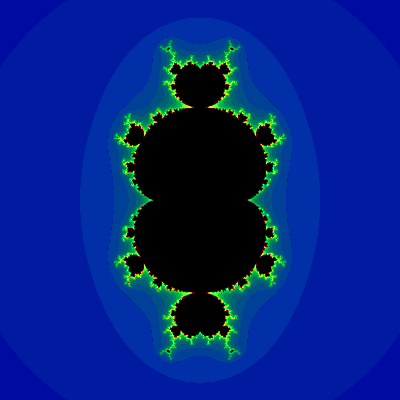Power of 4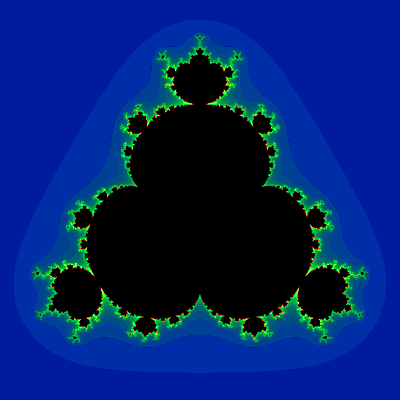Power of 5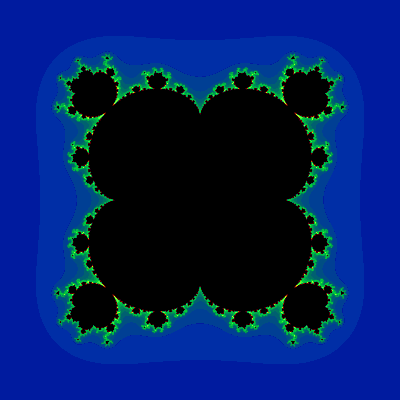Power of 6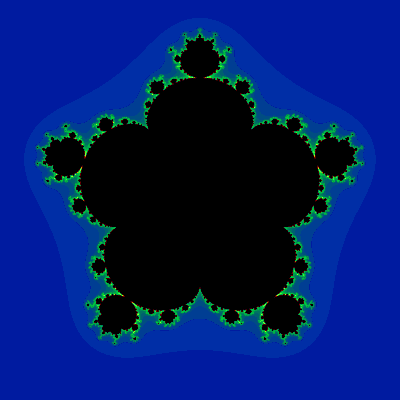With this function, you can actually generate these sets

and with a bit of modification to the function, you can get very detailed

and yes, even with different powers

How the function determines if something is in or out of the set, if checking if the real part of z to the power of 2 plus the imaginary part to the power of 2 is less than 4, if it is than its apart of the set, else, it isnt

Example:

``````local
local x = complex.mandelbrot({0,1},2) -- x = true
local y = complex.mandelbrot({0,1},4) -- x = false
local z = complex.mandelbrot({-2,0}) -- x = true

``````

This library also contains many math library functions now are compatible with output and input of complex numbers, such as:

• Sine (sin)
• Cosine (cos)
• Tangent (tan)
• Arcsine (asin)
• Arccosine (acos)
• Arctangent (atan)
• Sine Hyperbolic (sinh)
• Cosine Hyperbolic (cosh)
• Tangent Hyperbolic (tanh)
• Logarithm - The base and argument can be complex and/or negative (log)
• Power (pow)
• Square Root (sqrt)

And also makes some normal functions compatible with complex numbers

• Nth Fibonacci Number (fibonacci)
• Summation/Product (summation/product)
• Vertex Calculator (vertex)
• Tetration (tetration)

## Constants

Some mathematical constants that are important (not in programming)

Euler's Constant

Purpose: return the number e

Output: Number
Explanation:

Example:

``````local e = module.e -- e = 2.718281828459045
``````
The Golden Ratio

Purpose: return the number phi

Output: Number
Explanation:

Example:

``````local phi  = module.phi -- phi  = 1.618033988749895
``````
Pi

Purpose: return the number pi

Output: Number
Explanation:

Example:

``````local pi  = module.pi -- pi  = 3.141592653589793
``````
Tau

Purpose: return the number tau

Output: Number
Explanation:

• All tau is is 2×pi

Example:

``````local tau = module.tau -- tau = 6.283185307179586
``````
Message From Developer

This may not concern anyone whos just here for the module, however, if you’ve taken notice of another module already under the name MathAddons, and to put into words, I am the same person, I’ve been terminated for unfair reasons. I won’t go into the depth of the ban, I am only trying to clear up any confusion.

This module is a complete remake of its predecessor, and contains 50 functions, while the previous one only contained 26. The previous module (obviously) won’t be receiving updates.

(Note that some functions that perform the same task, may have different parameters and/or different names, do make sure you are making the necessary changes to your code of you are switching)

If you have any issues or critiques about the module please leave a reply, I’m currently a student whos only taken the Algebra I class (recieved a 94 on the final!!), so if I incorrectly explained anything (especially in the Calculus category), please get my attention!

57 Likes

Wow! This is insane, Great job!

4 Likes

But how is this useful, in a big way
I can just use a calculator

1 Like

You’re right about it being a lot, there’s a lot to document. You may use a calculator if you wish, ive mentioned that working on this module is mainly a hobby of mine, I barely thought of applicability when creating this.

3 Likes

The summation goes in a loop. I remember this other algorithm that did not require loops. It might cause a slight change but in a huge number it will be a huge improvement.

Your code uses a function argument, so I am not sure if it will work with that.

This will be useful for me, good job working on this!

1 Like

Typo, I think you mean Lucas sequence.

1 Like

This module looks really useful! I would totally use this for later projects of mine or maybe math homework, thank you for releasing this!

1 Like

One of the best module that i’ve seen. 10/101 Like

You’re right about there being an algorithm, I’ll try and figure out a way to use it with functions.

This is insanely useful. Thanks so much! Can I get the source code, I need to study for my math testJK LOL

3 Likes

Really useful for my simulator game! Great Job!

1 Like

The examples use the wrong functions. Maximum uses `module.min`

1 Like

Thank you for reporting! I overlooked that when copying between the minimum and maximum category.

Yeah its not only minimum and maximum, I remember multiple being like that. For example range uses the iqr function.

Update 1 - Imaginary Number Library
Sunday, June 19th, 2022

# New Functions

## Statistics & Probability

Linear Regression

Purpose: returns the function (or slope & y intercept) and correlation coefficient of a data set
Parameters:

1. As many data points as you please in the form of {x,y}
2. The last parameter would be a boolean to Toggle Function Format

Output: Function, Number (If Function Toggle is false: Number, Number, Number
Explanation:

• *Calculates the line of best fit, and the correlation coefficient (the number that represents how good the line matches the set.
Example:
``````local slope, yint, r = module.linreg({0,2},{1,2},{2,3},{5,9},{6,5},{4,7},{10,5})
-- slope = 0.4142857142857143
-- yint = 3.057142857142857
-- r = 0.5385164807134504
local func, r = module.linreg({1,2},{5,8},{4,5},true)
-- r = 0.9607689228305227
local predictedPoint = func(10) -- predictedPoint = 14.23076923076923
``````

# New Category

## Imaginary Library

These functions assist you when working with arithmetic on complex numbers. It gets unreal.
Before the you read the documentation below, do note what a valid complex number is:

``````local complexNum = {1,2} -- This represents 1+2i
local realNum = 2 -- this represents 2+0i, this is a valid input, same thing as {2,0}
local imaginaryNum = {0,5} -- this represents 0+5i, this is a valid input
local invalidinput = {5} -- this will return an error
local complex = module.Complex -- this is how you call the library
``````

Note that most of these functions require an input of 2 tables, with two values each

Purpose: combine two complex numbers
Parameters:

1. Table - {a,b}
2. Table - {c,d}
3. String Format Toggle (OPTIONAL) || Defaulted to false

Output: Table/String
Explanation:

• (a+bi)+(c+di) = (a+c)+(b+d)i

Example:

``````local
local x = complex.add({2,1},{0,3},true) -- x = '2+4i'
local y = complex.add({-5,1},4) -- y = {-1,1}
``````
Multiplication

Purpose: multiply two complex numbers
Parameters:

1. Table - {a,b}
2. Table - {c,d}
3. String Format Toggle (OPTIONAL) || Defaulted to false

Output: Table/String
Explanation:

• (a+bi)(c+di), you just distribute the binomial, anywhere you see an i^2, it becomes a -1 = ac-bd+(ad+cd)i

Example:

``````local
local x = complex.mul({2,1},{0,3},true) -- x = '-3+6i'
local y = complex.mul({-5,1},4) -- y = {-20,4}
``````
Division

Purpose: divide two complex numbers
Parameters:

1. Table - {a,b}
2. Table - {c,d}
3. String Format Toggle (OPTIONAL) || Defaulted to false

Output: Table/String
Explanation:

• (a+bi)/(c+di) multiply both sides by the conjugate as the numerator and denominator. you get (a+bi)(c-di)/(c+di)(c-di), which simplifies to (ac+bd+(bc-ad)i)/(c^2+d^2)

Example:

``````local
local x = complex.div({2,1},{4,3},true) -- x = '0.44+-0.08i'
local y = complex.div({-5,1},4) -- y = {-1.25,.25}
``````
To Polar Form

Purpose: convert a rectangular value to polar
Parameters:

1. Table - {a,b}
2. String Format Toggle (OPTIONAL) || Defaulted to false

Output: Number, Number
Explanation:

• converting a rectangular form value to polar requires you to find the distance from the origin of the imaginary axis is that point: sqrt(a^2+b^2), and the angle if there was a line to be drawn from the origin to the point is the arctan(b/a)

Example:

``````local
local x = complex.toPolar({2,1},true) -- x = '2.23606797749979e^0.4636476090008061i'
local distance,theta = complex.toPolar({-5,1})
-- distance = 5.0990195135927845,
-- theta = -0.19739555984988078
``````
From Polar Form

Purpose: convert a rectangular value to polar
Parameters:

1. Number - Distance
2. Number - Angle
3. String Format Toggle (OPTIONAL) || Defaulted to false

Output: Table/String
Explanation:

• Converting polar form into rectangular form requires you to convert from polar form to Euler’s identity (re^iθ = r×cos(θ) + r×isin(θ), which can just be calculated using trig functions and arithmetic

Example:

``````local
local x = complex.fromPolar(2.23606797749979,0.4636476090008061,true) -- x = '2+1i'
local y = complex.fromPolar(5.0990195135927845,0.19739555984988078) -- y = {-5,1}
``````
Exponentiation

Purpose: set a complex number to a power of a complex number
Parameters:

1. Table - {a,b}
2. Table - {c,d}
3. String Format Toggle (OPTIONAL) || Defaulted to false

Output: Table/String
Explanation:

• Too tired to explain how this works, go check out the source code

Example:

``````local
local x = complex.pow({0,1},{0,1},true) -- x = '0.20787957635+0i' (This is actually i^i)
local y = complex.pow(3,3,true) -- y = '27+0i' (just 3^3)
local z = complex.pow({0,1},{2,0},true) -- z = '-1+0i' (this is i^2 which is defined as -1'
local a = complex.pow(-1,.5,true) -- a = '-1+0i' (this is i which is defined as sqrt(-1)', note that with powers less than 1, its important to know that there are more than 1 solutions to these powers, the module has to decide on 1)
``````
Mandelbrot Checker

Purpose: Check if a complex number is in the mandelbrot set
Parameters:

1. Table - {a,b}
2. Number - Power (OPTIONAL) || Defaulted to 2

Output: Boolean
Explanation:

• This requires a bit of representation
Images

This below is the mandelbrot set

everything inside of it is a solution, so inputing any complex number inside the set will return true
What is the second parameter you ask?
Well, the mandelbrot set is generated by iterating this function an infinity amount of times
z = 0
z = z^2+c
where the z starts at 0 and c is the input. then z is equal to whatever the solution to 0^2+c is, and you repeat the process of subsituting whatever the output was back into z.
The second parameter is the power, you can tell in the equation that the set has the highest power of 2, the second parameter changes that number
Power of 3Power of 4Power of 5Power of 6With this function, you can actually generate these sets

and with a bit of modification to the function, you can get very detailed

and yes, even with different powers

How the function determines if something is in or out of the set, if checking if the real part of z to the power of 2 plus the imaginary part to the power of 2 is less than 4, if it is than its apart of the set, else, it isnt

Example:

``````local
local x = complex.mandelbrot({0,1},2) -- x = true
local y = complex.mandelbrot({0,1},4) -- x = false
local z = complex.mandelbrot({-2,0}) -- x = true

``````

# Documentation Fixes

• Some mistakes in the example code such as incorrect function names, thanks to
@Dev98799 for bringing this to my attentionThank you for all the support on this module2 Likes

Wow, this looks really useful, and will definitely consider installing it in my game!

1 Like

this my good sir is what i would call a banger

good stuff!!! super proud1 Like

Man that is pretty cool. I do not even think that the calculus functions are useless. In fact I was able to approximate pi up to 7 decimal digits correct with just three lines of code :

``````local Math = require(game.ReplicatedStorage.MathAddons)

s = 2 * Math.integral(-1,1, function(x) return math.sqrt(1-x^2) end)
print(s)
``````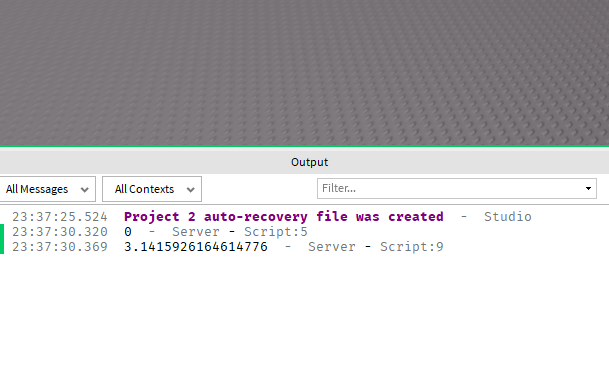For anyone interested in math here is why this works:

Assume we have a circle with radius 1. Its area is then the following: pi * r^2 = pi * 1 = pi
So, by knowing the area of such a circle we can theoretically calculate pi. We also know that there is a function that describes the curve of the half of the circle which in our case is the following:

Thus if we can find the area of the region between the points -1 and 1 and multiply it by two we can then find the area of our whole circle which in our case is equal to pi. How can we do this? Luckily for us there is the first fundamental theorem of calculus which states that

Let f(x) be a continuous positive function between a and b and consider the region below the curve y = f(x), above the x-axis and between the vertical lines x = a and x = b. Then denote the area of this region by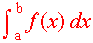and call this the definite integral of f(x) from a to b.

We know our function which is y=f(x)=sqrt(1-x^2) and our points a and b are -1 and 1 respectively. Thus the area under the curve is :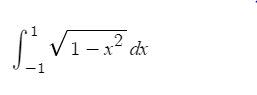The problem with this though is that the indefinite integral of sqrt(1-x^2) is the following: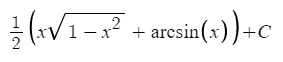Now, you will ask what is the problem with the above? The answer is that the arcsin formula is the following:
arcsin(x) = π/2 - arccos(x) So as you understand we can not find pi using pi because we will end in something like this 0=0. Now, you might ask, how did the above module calculate pi ? The answer is that it did not calculate it, but it approximated it with the following method:

As you can see, the integral function does the following things:
First it makes sure that the parameters are in fact two numbers and a function. Then it checks whether the upper bound is less than zero and if it is, it takes the absolute value of it. In our case the upper bound is equal to one so there is no need to take its absolute value. Next, it does the following thing:

s = sqrt(1-(-1))^2 * 1e-5 + sqrt(1-1^2)*1e-5 + sqrt(1-(1e-5)^2)*1e-5 which then becomes
s = 0 + 0 + sqrt(1-(1e-5)^2) * 1e-5 which when multiplied by two is an approximation of our area and hence of pi.
I have to admit that the methods that are used in both calculus functions are smart because in reality these seemingly random numbers (1e-12) and (1e-5) are small changes(which is exactly what calculus examines, changes) that will approximate the result.

2 Likes

Great work, mate! Would you consider adding a function to convert a string to an equation and an equation to a string?

Input: “3 + 3” OR 3 + 3
Output: 3 + 3 OR “3 + 3”

2 Likes